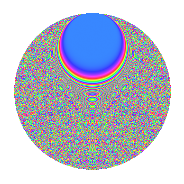# Properties

 Label 2015.2.giLevel 2015 Weight 2 Character orbit gi Rep. character $$\chi_{2015}(141,\cdot)$$ Character field $$\Q(\zeta_{60})$$ Dimension 2400 Sturm bound 448

# Related objects

## Defining parameters

 Level: $$N$$ = $$2015 = 5 \cdot 13 \cdot 31$$ Weight: $$k$$ = $$2$$ Character orbit: $$[\chi]$$ = 2015.gi (of order $$60$$ and degree $$16$$) Character conductor: $$\operatorname{cond}(\chi)$$ = $$403$$ Character field: $$\Q(\zeta_{60})$$ Sturm bound: $$448$$

## Dimensions

The following table gives the dimensions of various subspaces of $$M_{2}(2015, [\chi])$$.

Total New Old
Modular forms 3648 2400 1248
Cusp forms 3520 2400 1120
Eisenstein series 128 0 128

## Trace form

 $$2400q + 36q^{6} - 20q^{7} + 72q^{8} - 304q^{9} + O(q^{10})$$ $$2400q + 36q^{6} - 20q^{7} + 72q^{8} - 304q^{9} + 8q^{11} - 16q^{12} + 20q^{13} - 64q^{14} - 312q^{16} + 24q^{17} + 52q^{18} + 20q^{19} - 8q^{20} - 200q^{21} - 24q^{22} + 80q^{28} + 24q^{29} - 8q^{31} - 128q^{32} - 32q^{33} - 72q^{34} + 68q^{37} - 92q^{39} + 4q^{41} - 192q^{43} - 20q^{44} - 16q^{45} - 88q^{46} + 48q^{47} - 24q^{51} + 224q^{52} + 72q^{53} - 376q^{57} - 96q^{58} - 40q^{59} - 72q^{61} - 96q^{62} + 20q^{63} - 540q^{64} + 48q^{66} + 60q^{67} + 72q^{68} - 360q^{69} + 8q^{70} + 96q^{71} - 44q^{72} - 88q^{73} + 8q^{75} + 240q^{76} + 76q^{78} - 32q^{79} - 96q^{80} + 588q^{81} + 120q^{82} - 12q^{83} - 128q^{84} - 168q^{86} - 304q^{87} + 120q^{88} + 268q^{89} - 48q^{91} - 128q^{93} + 16q^{94} - 152q^{96} + 128q^{97} - 180q^{98} - 240q^{99} + O(q^{100})$$

## Decomposition of $$S_{2}^{\mathrm{new}}(2015, [\chi])$$ into irreducible Hecke orbits

The newforms in this space have not yet been added to the LMFDB.

## Decomposition of $$S_{2}^{\mathrm{old}}(2015, [\chi])$$ into lower level spaces

$$S_{2}^{\mathrm{old}}(2015, [\chi]) \cong$$ $$S_{2}^{\mathrm{new}}(403, [\chi])$$$$^{\oplus 2}$$